Introduction of energy performance assessment of lightning system:

Lighting is provided in industries, commercial buildings, indoor and outdoor for providing comfortable working environment. The primary objective is to provide the required lighting effect for the lowest installed load i.e highest lighting at lowest power consumption.

The purpose behind ‘Performance Test’:

Most interior lighting requirements are for meeting average luminance on a horizontal plane, either throughout the interior, or in specific areas within the interior combined with general lighting of lower value.

The purpose of performance test is to calculate the installed efficacy in terms of lux/watt/m² (existing or design) for general lighting installation. The calculated value can be compared with the norms for specific types of interior installations for assessing improvement options.

The installed load efficacy of an existing (or design) lighting installation can be assessed by carrying out a survey as indicated in the following pages.

Important Terms in Lightning

Lumen is a unit of light flow or luminous flux. The lumen rating of a lamp is a measure of the total light output of the lamp. The most common measurement of light output (or luminous flux) is the lumen. Light sources are labeled with an output rating in lumens.

Lux is the metric unit of measure for luminance of a surface. One lux is equal to one lumen per square meter.
Circuit Watts is the total power drawn by lamps and ballasts in a lighting circuit under assessment.

Installed Load Efficacy is the average maintained luminance provided on a horizontal work- ing plane per circuit watt with general lighting of an interior. Unit: lux per watt per square metre (lux/W/m²)

Lamp Circuit Efficacy is the amount of light (lumens) emitted by a lamp for each watt of power consumed by the lamp circuit, i.e. including control gear losses. This is a more meaningful measure for those lamps that require control gear. Unit: lumens per circuit watt (lm/W)

Installed Power Density – The installed power density per 100 lux is the power needed per square metre of floor area to achieve 100 lux of average maintained luminance on a horizontal working plane with general lighting of an interior. Unit: watts per square meter per 100 lux (W/m²/100 lux)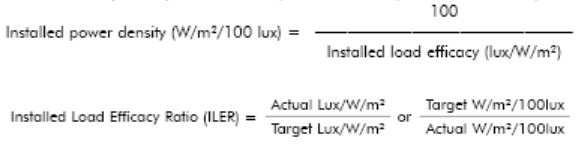Average maintained luminance is the average of lux levels measured at various points in a defined area.

Colour Rendering Index (CRI) is a measure of the effect of light on the perceived color of objects. To determine the CRI of a lamp, the colour appearances of a set of standard colour chips are measured with special equipment under a reference light source

Preparation before measurement

Before starting the measurements, the following care should be taken:

• All lamps should be operating and no luminaries should be dirty or stained.
• There should be no significant obstructions to the flow of light throughout the interior, especially at the measuring points.
• Accuracies of readings should be ensured by
– Using accurate luminance meters for measurements
– Sufficient number and arrangement of measurement points within the interior–Proper positioning of luminance meter
– Ensuring that no obstructions /reflections from surfaces affect measurement.

The interior is divided into a number of equal areas, which should be as square as possible. The luminance at the centre of each area is measured and the mean value calculated. This gives an estimate of the average luminance on the horizontal working plane.

Step by step procedure of lightning system

To Determine the Minimum Number and Positions of Measurement Points

Calculate the Room Index: RI = L x W/ Hm(L + W)
Where L = length of interior; W = width of interior; Hm = the mounting height, which is the height of the lighting fittings above the horizontal working plane. The working plane is usually assumed to be 0.75m above the floor in offices and at 0.85m above floor level in manufacturing areas.

Determination of Measurement Points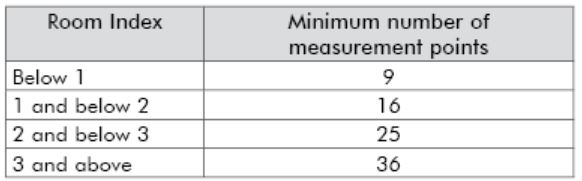To obtain an approximately ‘square array’, i.e. the spacing between the points on each axis to be approximately the same, it may be necessary to increase the number of points.

For example, the dimensions of an interior are:

Length = 9m, Width = 5m, Height of luminaires above working plane (Hm) = 2m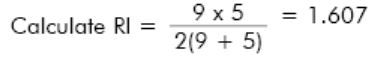From above Table the minimum number of measurement points is 16

As it is not possible to approximate a ‘square array’ of 16 points within such a rectangle it is necessary to increase the number of points to say 18, i.e. 6 x 3. These should be spaced as shown below:Therefore in this example the spacing between points along rows along the length of the interior = 9 ÷ 6 = 1.5m and the distance of the ‘end’ points from the wall = 1.5 ÷ 2 = 0.75m.

Similarly the distance between points across the width of the interior = 5 ÷ 3 = 1.67m with half this value, 0.83m, between the ‘end’ points and the walls.

In olden age, incandescence and fluorescence lamps were the main focus in illumination technology. With continuous development

Led: Principle of Operation

LEDs differ from traditional light sources in the way they produce light An LED, is a semiconductor diode. It consists of a chip of semiconducting material treated to create a structure called a pn junction.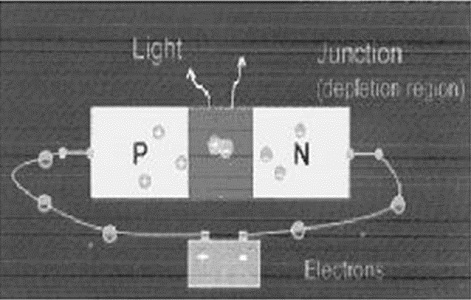When connected to a power source, current flows from the p-side (or anode) to the n-side (or cathode) and not in the reverse direction. The charge-carriers (electrons and electron holes) flow into the junction from electrodes. When an electron meets a hole, it falls into a lower energy level, and releases energy in the form of a photon (light). The specific wavelength or color emitted by the LED depends on the materials used to make the diode.

Recent Developments in Led Lighting

The efficacy of light source is measured in lumens/watt. The efficacy of LED is compatible with the present light source but the efficiency of LED lighting is very high. As in normal incandescence lamp is having efficacy of around 18 lumens/watts and LEDs are in the range of 40 Lumens/watt, but in incandescence lamps most of the power (watts) lost in heat as the efficiency of incandesce lamp is very low in the range of 10-15%. As there is no heat developed in LEDs this power towards heat will be reduced, only losses taking place will be in driver circuits which account for 10-15%losses, thus a higher efficiency in the range of 85-90 % can be obtained. That makes a potential difference in saving in energy in LED lighting. The research is going on in development of LED with high lumens/watt output. The maximum achieved efficacy is 132 lm/w, but it is yet to be commercialised. By passing a high current through a LED higher lumens/watt can be obtained with increase in power rating as well. Generally 1 w LEDs are considered high watt LED and are in use for illumination purpose.

Organic light-emitting diodes (OLED) can be a revolutionary change in display purpose. With development of OLED, it is possible to make LED displays as thin as paper. An electronic paper which can be folded and carried away. Such displays can be very useful for advertisement purpose.

Comparison of Leds with other type of light sources

As discussed earlier the efficacy of LEDs is not very high. Following chart shows the comparison of efficacy of various illumination schemes: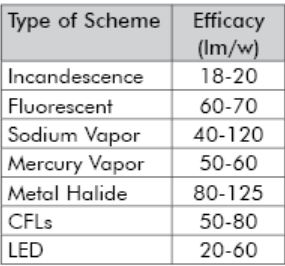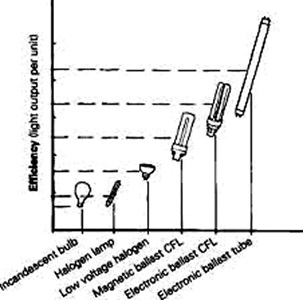From above table it can be observed that the efficacy of LEDs is on par with CFLs, but as the driver losses are negligible and there no production of heat, thus giving higher efficiency.

Case Study for the Energy Conservation Awareness

Case study – I of replacing conventional lighting system (fluorescent) by LEDS and/or CFL:

In an Engineering college there were 113 conventional tube lights, the tube lights use to ON from 8 PM to 6 PM (Timing use to vary subject to season change). The tube lights were of standard make.
After testing on choke it was observed that chokes are consuming 13 watts of power (average).
Thus total power loss using tube lights PLoss=113 x 13= 1469 watts.
The tube lights (on an average) working for 10 hours a day.
Total Energy loss per day = 14.69 kWH Financial Loss per day = 73/- @ 5/- per unit This amount for accounted per day only to make up the losses.
These fixtures were provided with 40 W tube lights.
Total power consumed with 40 W tube (for purpose of vigilance) =113 x 40 = 4520W
The financial burden = 4.52 x 10 x 5 = Rs. 226/-
Total Expenditure per day (towards vigilance) = 226 + 73 = RS. 300/-
In one year = Rs. 1, 09,500/-
i) If these lights were replaced with CFLs:
Lux obtained by a fluorescent Tube = 2200. The commercially available CFL of 15 W will provide a lux of 1000, so number of CFLs required to produce required lighting = 2 Power consumed by TWO CFLs = 30 Watts (53 watts in case of Tube lights).
NOTE: Though the lux produced by CFLs is less, it is sufficient enough to produce required illumination.
Power saved = 53 – 30 = 23 Watts
Energy saved per day = 0.023 x 113 x 10 = 26 units per day
Financial saving = 26 x 5 = Rs.130/- per day.
Saving over one year = 130 x 365 = 47,450, Rs. 47,450/-
Investment (Initial cost) to replace Tubes with CFLs
Cost of one CFL = Rs. 110/-
Cost of fitting to fix CFL = Rs. 12/- Total of one CFL with fitting = Rs. 122/- No. of tubes to be replaced = 113.
Equivalent number of CFLs = 226 (@ Two CFLs per tube light)
Total Initial investment = 226 x 122 = Rs. 27,572/-
Payback period = 27572/47450 = 0.58 years =0.58 x 12 = 7 (Approx. seven months).
ii) If tube lights were replaced with LED lighting system:
The white color LEDs, in LED Pack form (24 LEDs per pack) manufactured by SECO Instruments Pvt Ltd are used.
Two LED systems of around 1.4 – 2 W each will produce a required illumination that produced by one tube light.
Total power consumed by two LED systems = 4 W
Power saved = 53-4 = 49 W
Energy saved per day = 0.049 x 113 x 10 = 55.37 units
Financial Saving / day = 55.37 x 5 = Rs. 277/- Per year saving = Rs.1,01,105/-
Cost of ONE LED system = Rs. 500/-
Total LED system required = 113 x 2 = 226 (@ 2 LED system per tube light)
Total cost of LED systems = 226 x 500 = 1, 13,000/-Lighting system Annual Saving Investment Pay back Period
Present Nil Nil Nil
CFL 47450 27,570 6 months
LED 1,01,1
05 1,13,000 14 months

Pay Back period = 1, 13,000/1, 01,105 = 1.12 Years = 14 months.
From above comparison it can be seen that by replacing the present system of conventional tube lights, energy saving potential is possible with CFLs and LEDs. The CFL system is seems to be have quicker pay back period. But the above analysis can be compared depends on the life of the Lighting system as well.
CFLs are having a life span of around 5000- 7000 hours. At the rate of 10 hours per day a CFL will not stand for more than two years. Thus an investment of Rs. 30,000/- is bound over two years. Whereas LED last for 75000 to 100000 hours. Taking 75000 hours life, a LED system will last for at least 10 years.
Comparing over 10 years: CFL system:
Saving in CFL system = Rs. 4,75,000/- Investment ( 5 times) = Rs. 1,32,000/-
Net Saving = Rs. 3, 43,000/-
Saving in LED system = 10, 00,000/- Investment (One time) = 1, 20,000/-
Nett Saving = 8, 80,000/-
It can be observed that CFL system is no way comparable with LED system, over a period of 10 years.
Note: Cost of technology is coming down and that of energy is increasing. The saving will be at higher side with increase in energy cost and investment will be at lower side with decrease in cost.

Case Study – II – about power saving in outdoor lighting at college campus

Presently, there is a following power consumption for outdoor lighting at one college.
1) Metal halide of 400Wattage rating: 12 nos.
2) Small halogen of 500Wattage rating: 10 nos.

In order to calculate the monthly power consumption and electricity bill for the present scenario if this outdoor lighting is remaining on during 6:30 pm to 6:30am daily. Considering the unit rate is 10 Rs for HT electricity plan.

Now, if we replace this lighting system by 100wattage LED light-4nos. and 70Wattage LED light-10nos. Calculate monthly power consumption and electricity bill for the same case.This lighting will remain on during 6:30 pm to 6:30am daily. Consider the unit rate is 10 Rs for HT electricity plan . If the rate of 100Wattage LED light is 3101.85 rs/piece and 70 Wattage LED light is 2212.96 Rs/piece. We can calculate payback period for the LED lights. Consider no any maintenance for LED fittings. Guaranty is 2 years for LED lights.

Here,
Total Energy used per day by Lightning load is: ((12*400) + (10*500)) * 12
= 9800 *12 = 117600 Wh = 117.6 Units of power.
Total Cost per day = 1176 Rs.
Monthly cost = 1176 * 30 = 35280/- Rs.
Now, if 100 W- LED bulbs = 4 Nos.
And 70W LED bulbs = 10 Nos are used, then
Monthly power consumption = 30*12 Hr (4*100+10*70)
= 396 Units
Monthly cost = 396 *10 = 3960 Rs only.
Now, considering Total cost of LED = 10 (2212.96) + 4 (3101.85)
= 34,537 Rs.
Total Payback period is = Total spending / saving per month.
= 34537 / (35280-3960) = 1.1027 Months = 1 month and two days

Case Study – III –Energy Conservation in classroom by lightning

If no. of tube lights = 9 Rating: 40 W.
If number of fans = 8, rating: 75 W.
Time of operation: 8:00 am to 6:00 pm (10 hours)
Tariff plan: 5.22 Rs/unit
Daily Expenses= 10 (9 *40 +8*75) * 5.22 = 48.920 = 50 Rs.
Monthly expenses = 1500 Rs.
Let, if 5 fans are installed as price of new fan =2360*5 = 11800/- Rs &
And 5 tube lights are replaced as price of new LED tube light = 900*5 = 4500/- Rs.
So, total installation cost = 11800+4500= 16300/- Rs.
Now, rating of new tube light = 20 W and
Rating of new fan (preferably BLDC based) = 50 W
Daily expenses = 10 (20*5+ 50*5) * 5.22 Rs = 18.2 Rs
Monthly expenses = 550 Rs. (approx).
We will consider some financial parameters which should be also analysed while lightning scheme is to be selected.

 SPB (Simple Pay –Back period):
Simple Payback Period (SPP) represents, as a first approximation; the time (number of years)
required to recover the initial investment (First Cost), considering only the Net Annual Saving:
The simple payback period is usually calculated as follows:
Simple payback period = Expenses / savings
= 16300/ 1500-550
= 17.15 months
= 1 and half year (17 months and 15 days).

 Internal Rate of Return
This method calculates the rate of return that the investment is expected to yield. The internal rate of return (IRR) method expresses each investment alternative in terms of a rate of return (a compound interest rate). The expected rate of return is the interest rate for which total discounted benefits become just equal to total discounted costs (i.e net present benefits or net annual benefits are equal to zero, or for which the benefit / cost ratio equals one). The criterion for selection among alternatives is to choose the investment with the highest rate of return.

The rate of return is usually calculated by a process of trial and error, whereby the net cash flow is computed for various discount rates until its value is reduced to zero.

The internal rate of return (IRR) of a project is the discount rate, which makes its net present value (NPV) equal to zero.

 Net Present Value

The net present value (NPV) of a project is equal to the sum of the present values of all the cash flows associated with it.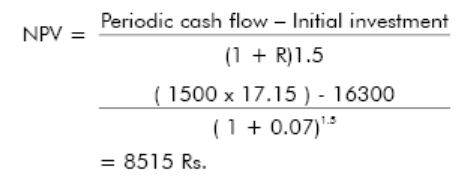Conclusion

In this article, authors have given the details of basics lightening about LED and explained the technical parameters of LED. Authors have also shown the case study for various lightning schemes. Some financial terms relevant to Lightning and Energy Conservation are also explained.